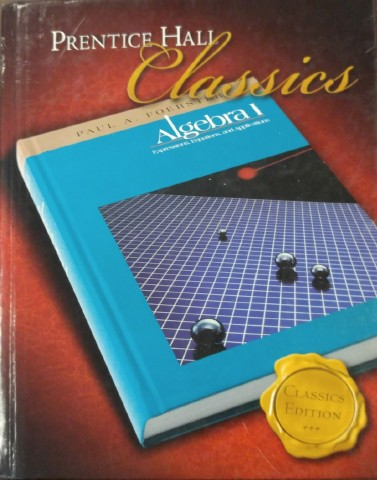7th Grade Advanced Algebra is a rigorous, fast-paced, first semester Algebra I course designed to prepare Academy 7th graders the rigors of the Accelerated Algebra I course that they will be taking in 8th grade.  Functions and fundamentals are the focus of this course.
The curriculum is planned and delivered to challenge the most capable math students.  In addition to the curriculum outlined in the textbook students will be exposed to some math topics that will not be studied in depth until future math and science courses.  Some time will be spent preparing for the SAT.
Appropriate use of technology (TI-84+) graphing calculator) will be introduced during the year, but it will be limited, and concentrated in the 2nd semester.  Every effort will be made to help students master the skills that will ensure that they are prepared to make a smooth transition to the math courses with the more sophisticated topics that they will encounter in the future.

This class will be taught using techniques that are being called, "Blended Learning".  This means that some homework requires students to watch a video that is the lesson for the latest topic.  They are expected to learn the material in the videos in the same way that they are expected to learn a lesson when it is given live, in class.  Blended Learning techniques have been shown to be at least as effective as in-person lesson presentations and have been incorporated in this class the past 2 years with great results.Textbook Overview:

The first 6 chapters (the part of the book we will complete in 7th grade) consist of a tightly-sequenced series of topics leading as quickly as possible to a mathematically honest derivation of the Quadratic Formula.  This departure from the traditional sequence of topics is made possible by using a calculator to evaluate radicals.
Chapter 5 introduces students to polynomials, including factoring.  This is done so that students have a solid grasp of how the process relates to completing the square.  One of the topics that will be presented later in the book will be introduced at end of Chapter 5.  That topic is Solving Quadratics by Factoring.
Time will be spent developing the concept of a variable as a symbol that represents a value that really varies, rather than simply standing for an unknown constant.  To aid in this development, the book presents mathematical expressions as something to which one does two things: evaluates, when the value of the variable is known, and finds the value of the variable when the value of the expression is known.  The problem solving in this book have multiple parts in which students must write an expression representing a variable quantity, then do these two things with the expression.  This process helps students to understand that a variable can stand for a different number at different times, depending on the situation.
The properties of numbers are first presented as verbs.  These are the Field Axioms where students learn how to Commute, Associate, etc.  Use of the axioms will require justification in order to give students an introduction to the idea of proofs (deductive reasoning).  The concept of Closure will also be introduced in a formal way so students can begin to better understand the properties of the Real Numbers.

The first trimester includes  lessons on SAT preparation.
There will be some topics introduced throughout the year that do not appear in the textbook.  They will be covered at the appropriate times when they naturally fit.

Chapter 1: Expressions and Equations;

1-1, Operations With Numbers--------------\
1-2, Variables                                               \
1-3, Powers and Exponents                            \
1-4, Order of Operations                                  \    Five weeks, with SAT prep.
1-5, Expressions From word Statements         /
1-6, Introduction to Equations                        /
1-7, Solving Equations                                   /
1-8, Problems That Lead to equations------/
1-9, Problems That Lead to Expressions and Equations (will be taught
using function language.)
1-10, Chapter Review

Chapter 2: Operations With Negative Numbers; this is a review of pre-algebra.

2-1, Intro. to Negative Numbers--------------------\
2-3, Subtracting Integers                                         \
2-4, Multiplying Integers                                          \    Five weeks, with SAT prep.
2-5, Dividing Integers                                               /
2-7, Equations That Need Two Transformations-/
2-8, Problems That Lead to Two Transformations.
2-9, Chapter Review

Chapter 3: Distributing Axioms and Other Properties; this is a pre-algebra review.

3-1, Distributing-------------------------------------------\
3-2, Distributing Multiplication Over Subtractio    \
3-3, More Distributive Properties                            \
3-4, Like Terms and common Factors                       \    Five weeks, with SAT prep.
3-5, Axioms for Adding & Multiplying                      /
3-6, Properties of Equality                                       /
3-7, Chapter Review-------------------------------------/

Chapter 4: Harder Equations; this is a pre-algebra review.

4-1, Equations With Like Terms----------------------\
4-2, Equations With Like Terms and Distributing \
4-3, Equations With Variables in Both Members   \    Five weeks, with Stanford prep.
4-4, Equations That Involve Decimals                      /
4-5, Literal Equations and Formulas------------------/
4-6, Problems That Involve More Than One Expression.
4-7, Chapter Review

------------------------------  Chapter 5 begins polynomials -----------------------------

Chapter 5: Some Operations With Polynomials and Radicals.

5-1, Introduction to Polynomials--------------------\
5-2, Names of Polynomials                                    \
5-3, Products of Two Binomials                               \
5-5, Factoring Quadratic Trinomials - 3rd Term Neg.
5-6, Factoring Quadratic Trinomials, a   1                /     Six weeks, with Stanford prep.
5-7, Factoring the Difference of Two Squares        /
5-8, Squaring a Binomial                                        /
5-9, Factoring Trinomial Squares                         /
5-10, Radicals, Irrationals, and Closure            /
5-11, Chapter Review-------------------------------/

6-3, Equations Containing Absolute Value \
6-4, Equations With Squares                        \
6-5, Equations With Trinomial Squares        \
6-6, Completing the square                           /     Six weeks.